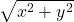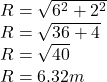## A man travels a distance if 1.5 m towards east ,then 2m towards south and 4.5 m towards east. i) What is the total distance travelle

Question

A man travels a distance if 1.5 m towards east ,then 2m towards south and 4.5 m towards east.
i) What is the total distance travelled
ii) what is the result in displacement

in progress 0
3 weeks 2021-09-04T20:39:45+00:00 1 Answers 0 views 0

## ii) 6.32m

Explanation:

i) The total distance traveled will be the sum total of individual distances traveled by the man.

If the man travels a distance if 1.5 m towards east ,then 2m towards south and 4.5 m towards east, the total distance traveled will be expressed as; 1.5m+2m+4.5m

= 8.0m

ii) In order to get the man resultant displacement, we will use the Pythagoras theorem. Since the man travels a distance if 1.5 m and 4.5m towards east, the total distance taken towards east will be 6.0m and the distance traveled towards south is 2.0m. The resultant displacement of the man is expressed as;

R =Note that the eastward direction is the x-direction while the south direction is the y-direction.

x = 6.0m and y = 2.0mThe displacement of the man is 6.32m